Download Presentation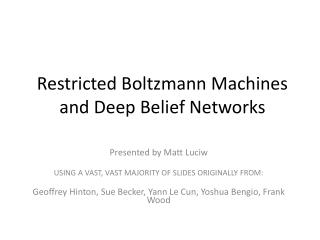Restricted Boltzmann Machines and Deep Belief Networks

# Restricted Boltzmann Machines and Deep Belief Networks - PowerPoint PPT Presentation

Restricted Boltzmann Machines and Deep Belief Networks. Presented by Matt Luciw USING A VAST, VAST MAJORITY OF SLIDES ORIGINALLY FROM: Geoffrey Hinton, Sue Becker, Yann Le Cun , Yoshua Bengio , Frank Wood . Motivations.I am the owner, or an agent authorized to act on behalf of the owner, of the copyrighted work described.
Download Presentation## Restricted Boltzmann Machines and Deep Belief Networks

An Image/Link below is provided (as is) to download presentation

Download Policy: Content on the Website is provided to you AS IS for your information and personal use and may not be sold / licensed / shared on other websites without getting consent from its author.While downloading, if for some reason you are not able to download a presentation, the publisher may have deleted the file from their server.

- - - - - - - - - - - - - - - - - - - - - - - - - - E N D - - - - - - - - - - - - - - - - - - - - - - - - - -
Presentation Transcript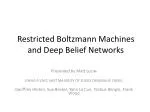### Restricted Boltzmann Machines and Deep Belief Networks

Presented by Matt Luciw

USING A VAST, VAST MAJORITY OF SLIDES ORIGINALLY FROM:

Geoffrey Hinton, Sue Becker, Yann Le Cun, YoshuaBengio, Frank Wood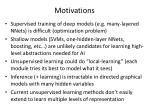Motivations
• Supervised training of deep models (e.g. many-layered NNets) is difficult (optimization problem)
• Shallow models (SVMs, one-hidden-layer NNets, boosting, etc…) are unlikely candidates for learning high-level abstractions needed for AI
• Unsupervised learning could do “local-learning” (each module tries its best to model what it sees)
• Inference (+ learning) is intractable in directed graphical models with many hidden variables
• Current unsupervised learning methods don’t easily extend to learn multiple levels of representation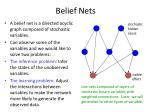A belief net is a directed acyclic graph composed of stochastic variables.

Can observe some of the variables and we would like to solve two problems:

The inference problem: Infer the states of the unobserved variables.

The learning problem: Adjust the interactions between variables to make the network more likely to generate the observed data.

Belief Nets

stochastic

hidden cause

visible

effect

Use nets composed of layers of stochastic binary variables with weighted connections. Later, we will generalize to other types of variable.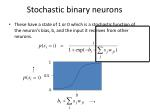Stochastic binary neurons
• These have a state of 1 or 0 which is a stochastic function of the neuron’s bias, b, and the input it receives from other neurons.

1

0.5

0

0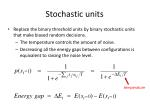Stochastic units
• Replace the binary threshold units by binary stochastic units that make biased random decisions.
• The temperature controls the amount of noise.
• Decreasing all the energy gaps between configurations is equivalent to raising the noise level.

temperature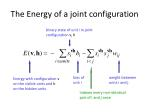The Energy of a joint configuration

binary state of unit i in joint configuration v, h

weight between units i and j

bias of unit i

Energy with configuration v on the visible units and h on the hidden units

indexes every non-identical pair of i and j once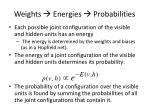Weights  Energies  Probabilities
• Each possible joint configuration of the visible and hidden units has an energy
• The energy is determined by the weights and biases (as in a Hopfield net).
• The energy of a joint configuration of the visible and hidden units determines its probability:
• The probability of a configuration over the visible units is found by summing the probabilities of all the joint configurations that contain it.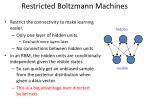Restricted Boltzmann Machines

hidden

• Restrict the connectivity to make learning easier.
• Only one layer of hidden units.
• Deal with more layers later
• No connections between hidden units.
• In an RBM, the hidden units are conditionally independent given the visible states.
• So can quickly get an unbiased sample from the posterior distribution when given a data-vector.
• This is a big advantage over directed belief nets

j

i

visible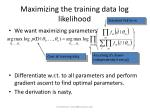Maximizing the training data log likelihood

Standard PoE form

• We want maximizing parameters
• Differentiate w.r.t. to all parameters and perform gradient ascent to find optimal parameters.
• The derivation is nasty.

Assuming d’s drawn independently from p()

Over all training data.

Frank Wood - fwood@cs.brown.edu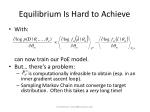Equilibrium Is Hard to Achieve
• With:

can now train our PoE model.

• But… there’s a problem:
• is computationally infeasible to obtain (esp. in an inner gradient ascent loop).
• Sampling Markov Chain must converge to target distribution. Often this takes a very long time!

Frank Wood - fwood@cs.brown.eduA very surprising fact
• Everything that one weight needs to know about the other weights and the data in order to do maximum likelihood learning is contained in the difference of two correlations.

Expected value of product of states at thermal equilibrium when the training vector is clamped on the visible units

Expected value of product of states at thermal equilibrium when nothing is clamped

Derivative of log probability of one training vectorThe (theoretical) batch learning algorithm
• Positive phase
• Clamp a data vector on the visible units.
• Let the hidden units reach thermal equilibrium at a temperature of 1
• Sample for all pairs of units
• Repeat for all data vectors in the training set.
• Negative phase
• Do not clamp any of the units
• Let the whole network reach thermal equilibrium at a temperature of 1 (where do we start?)
• Sample for all pairs of units
• Repeat many times to get good estimates
• Update each weight by an amount proportional to the difference in in the two phases.Solution: Contrastive Divergence!
• Now we don’t have to run the sampling Markov Chain to convergence, instead we can stop after 1 iteration (or perhaps a few iterations more typically)
• Why does this work?
• Attempts to minimize the ways that the model distorts the data.

Frank Wood - fwood@cs.brown.eduContrastive Divergence
• Maximum likelihood gradient: pull down energy surface at the examples and pull it up everywhere else, with more emphasis where model puts more probability mass
• Contrastive divergence updates: pull down energy surface at the examples and pull it up in their neighborhood, with more emphasis where model puts more probability massRestricted Boltzmann Machines
• In an RBM, the hidden units are conditionally independent given the visible states. It only takes one step to reach thermal equilibrium when the visible units are clamped.
• Can quickly get the exact value of :

hidden

j

i

visiblej

j

j

j

a fantasy

i

i

i

i

t = 0 t = 1 t = 2 t = infinity

Start with a training vector on the visible units.

Then alternate between updating all the hidden units in parallel and updating all the visible units in parallel.Contrastive divergence learning: A quick way to learn an RBM

j

j

Start with a training vector on the visible units.

Update all the hidden units in parallel

Update the all the visible units in parallel to get a “reconstruction”.

Update the hidden units again.

i

i

t = 0 t = 1

reconstruction

data

This is not following the gradient of the log likelihood. But it works well.

When we consider infinite directed nets it will be easy to see why it works.How to learn a set of features that are good for reconstructing images of the digit 2

50 binary feature neurons

50 binary feature neurons

Decrement weights between an active pixel and an active feature

Increment weights between an active pixel and an active feature

16 x 16 pixel image

16 x 16 pixel image

data (reality)

reconstruction (better than reality)Using an RBM to learn a model of a digit class

Reconstructions by model trained on 2’s

Data

Reconstructions by model trained on 3’s

100 hidden units (features)

j

j

256 visible units (pixels)

i

i

reconstruction

dataThe final 50 x 256 weights

Each neuron grabs a different feature.How well can we reconstruct the digit images from the binary feature activations?

Reconstruction from activated binary features

Reconstruction from activated binary features

Data

Data

New test images from the digit class that the model was trained on

Images from an unfamiliar digit class (the network tries to see every image as a 2)### Deep Belief NetworksExplaining away (Judea Pearl)
• Even if two hidden causes are independent, they can become dependent when we observe an effect that they can both influence.
• If we learn that there was an earthquake it reduces the probability that the house jumped because of a truck.

-10

-10

truck hits house

earthquake

posterior

20

20

p(1,1)=.0001

p(1,0)=.4999

p(0,1)=.4999

p(0,0)=.0001

-20

house jumpsProblem 1: The posterior is typically intractable because of “explaining away”.

Problem 2: The posterior depends on the prior created by higher layers as well as the likelihood.

So to learn W, we need to know the weights in higher layers, even if we are only approximating the posterior. All the weights interact.

Problem 3: We need to integrate over all possible configurations of the higher variables to get the prior for first hidden layer. Yuk!

Why multilayer learning is hard in a sigmoid belief net.

hidden variables

hidden variables

prior

hidden variables

likelihood

W

dataSolution: Complementary Priors
• There is a special type of multi-layer directed model in which it is easy to infer the posterior distribution over the hidden units because it has complementary priors.
• This special type of directed model is equivalent to an undirected model.
• At first, this equivalence just seems like a neat trick
• But it leads to a very effective new learning algorithm that allows multilayer directed nets to be learned one layer at a time.
• The new learning algorithm resembles boosting with each layer being like a weak learner.Infinite DAG with replicated weights.

An ancestral pass of the DAG is exactly equivalent to letting a Restricted Boltzmann Machine settle to equilibrium.

This infinite DAG defines the same distribution as an RBM.

An example of a complementary prior

etc.

h2

v2

h1

v1

h0

v0The variables in h0 are conditionally independent given v0.

Inference is trivial. We just multiply v0 by

This is because the model above h0 implements a complementary prior.

Inference in the DAG is exactly equivalent to letting a Restricted Boltzmann Machine settle to equilibrium starting at the data.

Inference in a DAG with replicated weights

etc.

h2

v2

h1

v1

h0

v0Divide and conquer multilayer learning
• Re-representing the data: Each time the base learner is called, it passes a transformed version of the data to the next learner.
• Can we learn a deep, dense DAG one layer at a time, starting at the bottom, and still guarantee that learning each layer improves the overall model of the training data?
• This seems very unlikely. Surely we need to know the weights in higher layers to learn lower layers?Multilayer contrastive divergence
• Start by learning one hidden layer.
• Then re-present the data as the activities of the hidden units.
• The same learning algorithm can now be applied to the re-presented data.
• Can we prove that each step of this greedy learning improves the log probability of the data under the overall model?
• What is the overall model?First learn with all the weights tied

This is exactly equivalent to learning an RBM

Contrastive divergence learning is equivalent to ignoring the small derivatives contributed by the tied weights between deeper layers.

Learning a deep directed network

etc.

h2

v2

h1

v1

h0

h0

v0

v0Then freeze the first layer of weights in both directions and learn the remaining weights (still tied together).

This is equivalent to learning another RBM, using the aggregated posterior distribution of h0 as the data.

etc.

h2

v2

h1

v1

v1

h0

h0

v0The RBM at the top can be viewed as shorthand for an infinite directed net.

When learning W1 we can view the model in two quite different ways:

The model is an RBM composed of the data layer and h1.

The model is an infinite DAG with tied weights.

After learning W1 we untie it from the other weight matrices.

We then learn W2 which is still tied to all the matrices above it.

A simplified version with all hidden layers the same size

h3

h2

h1

dataWhy greedy learning works
• Each time we learn a new layer, the inference at the layer below becomes incorrect, but the variational bound on the log prob of the data improves (only true in theory -ml).
• Since the bound starts as an equality, learning a new layer never decreases the log prob of the data, provided we start the learning from the tied weights that implement the complementary prior.
• Now that we have a guarantee we can loosen the restrictions and still feel confident.
• Allow layers to vary in size.
• Do not start the learning at each layer from the weights in the layer below.Why the hidden configurations should be treated as data when learning the next layer of weights
• After learning the first layer of weights:
• If we freeze the generative weights that define the likelihood term and the recognition weights that define the distribution over hidden configurations, we get:
• Maximizing the RHS is equivalent to maximizing the log prob of “data” that occurs with probabilityFine-tuning with a contrastive version of the “wake-sleep” algorithm

After learning many layers of features, we can fine-tune the features to improve generation.

1. Do a stochastic bottom-up pass

• Adjust the top-down weights to be good at reconstructing the feature activities in the layer below.
• Do a few iterations of sampling in the top level RBM

-- Adjust the weights in the top-level RBM.

• Do a stochastic top-down pass
• Adjust the bottom-up weights to be good at reconstructing the feature activities in the layer above.
• Not required! But helps the recognition rate (-ml).A neural network model of digit recognition

The top two layers form a restricted Boltzmann machine whose free energy landscape models the low dimensional manifolds of the digits.

The valleys have names:

2000 top-level units

10 label units

500 units

The model learns a joint density for labels and images. To perform recognition we can start with a neutral state of the label units and do one or two iterations of the top-level RBM.

Or we can just compute the free energy of the RBM with each of the 10 labels

500 units

28 x 28 pixel imageLimits of the Generative Model

1. Designed for images where non-binary values can be treated as probabilities.

• Top-down feedback only in the highest (associative) layer.
• No systematic way to deal with invariance.
• Assumes segmentation already performed and does not learn to attend to the most informative parts of objects.